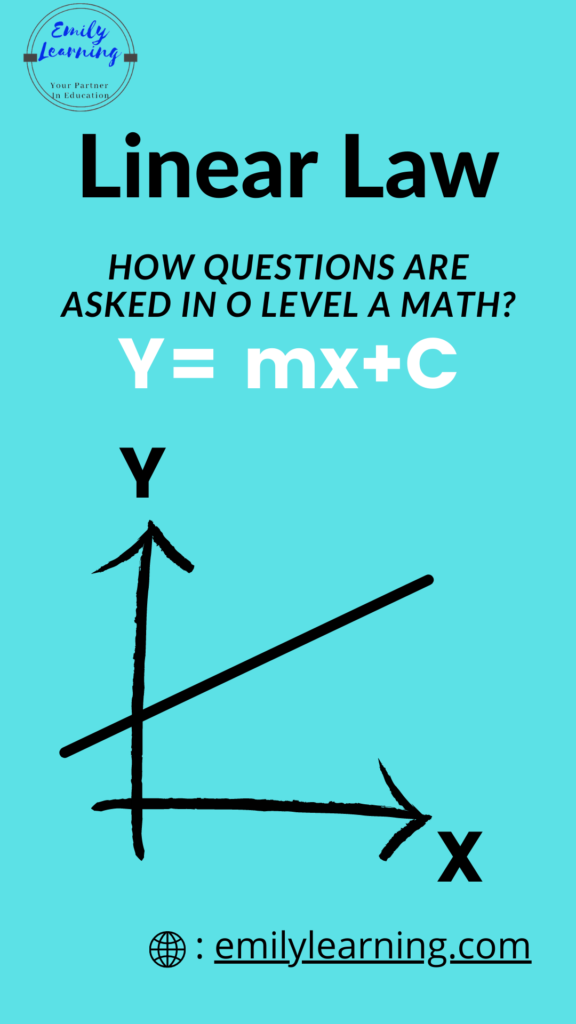# Linear Law A Math Questions are PredictableLinear Law questions in A Math are very predictable!

Because questions from this topic are predictable, it is easy to score for Linear Law O Level A Math once you are familiar with the structure.

Why are linear law questions highly predictable? Well, this is because questions on linear law are rather standard.

Each year, 1 question from this topic will come out in either paper 1 or paper 2.

In our previous posts, we talked about what is tested in this topic, and also go through a quick summary on the concepts tested in linear law.

In this post, let’s look at how a typical O Level A Math question on linear law is structured.

## Past year questions on Linear Law

Every year, there would be one question on linear law in O Level A Math Papers. Take a look at past questions on linear law.

Just by looking at the questions, you would notice that the questions are quite similar. This means that the questions from linear law chapter are predictable. Since you know what will be tested, it’s easy to score!

## What do most linear law questions require students to do?

Well, the first part of the question involves giving us a set of values of 2 variables. We need to manipulate some of the variables, and use these values to plot a straight line graph.

Once you have plotted a straight line graph, the next thing to do is to find the gradient and vertical intercept (or Y- intercept) so as to find the unknown constants.

Once this is found, we have formed a relationship between 2 (or more) variables. With that, the question will go on to ask us to find what is the value of one of the variable, given a certain value of another variable.

## Sample Question on Linear Law

I have provided a sample question on linear law, to go through step-by-step how to approach such questions. You can watch the video here. This is a sample from our course on linear law. To learn the entire chapter in detail, check out our A Math course on linear law here.

In this question, I go through step-by-step a typical linear law question. How to approach such a questions (which include finding vertical intercept and gradient), as well as the other questions that the O Level A Math examiners like to ask after you find the unknown constants.

To learn more on A Math, take a look at our other videos here.

## Learn On-demand – A- Math Courses

Want to learn A- Math on-demand? Check out O Level Additional Mathematics course here.

error: Content is protected !!